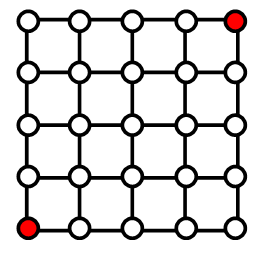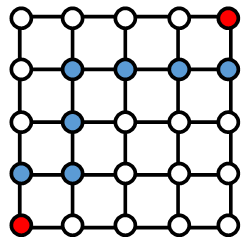# BBC micro:bit Lattic Paths

## Introduction

This is very much a niche topic but the page will include an outline of how you can use the code to make a game of sorts.

The idea came from solving a programming problem on the Project Euler web site. This site contains hundreds of programming challenges, some of them relatively simple, some incredibly challenging. Many programmers use these problems to develop and refine their programming skills or as a test drive for a new programming language.

Problem 15 on the site is about something called Lattice Paths and goes as follows,

Starting in the top left corner of a 2x2 grid, and only being able to move to the right and down, there are exactly 6 routes to the bottom right corner.How many such routes are there through a 20x20 grid?

Another way of representing the information from the problem is with a hierarchy chart, stating whether each step is to the south or to the east,As strings, we can write down all of the paths as follows,

EESS, ESES, ESSE, SEES, SESE, SSEE

The strings always consist of two steps to the south and two steps to the east. We could make all of the routes just by writing out all of the different ways we can make 2 S steps and 2 E steps. Imagine that you have 4 cards, 2 with an S, 2 with an E. You have 4 places to put them into to form the string. When you place your first card, there are 4 places to put it. When you place the second, there are now only 3 places left. 2 places left for the third and only one place left for your last one. That means,

There are 4 x 3 x 2 x 1 different ways that you can place your cards. That makes 24 different combinations. However, the cards are not all unique and duplicate strings will be created. Since we have two pairs of identical cards, we have to divide by 2 x 2 to get the number of unique strings.

This calculation can be written out as follows,And for the Euler problem,Finding an efficient way to perform that calculation is all that is needed for the problem.

## The micro:bit Part

So, what has this got to do with a micro:bit. Well, think of the LED matrix as a lattice, looking like this,Our task is to work out how to generate all of the valide lattice paths from the lit LED in the bottom left to the lit LED in the top right. The diagram shows us tha, since our LEDs mark the corners of the squares, our lattice is a 4x4 lattice.

Using the formula described above, we can find out that there are exactly 70 lattice paths to take us from one dot to another. Each path will involve eactly 8 steps. Since the last step is to an already lit LED, we will need to light 7 extra LEDS to show our paths.## Programming

If we want the program to calculate and know all of the possible routes, we need to think of a compact way of representing them. We have 8 steps which can be in one of two directions. This can be done with 8 bit binary numbers, where a 1 represents a step to the east and a 0 to the north. All of our numbers will need to contain exactly 4 1s and exactly 4 0s. We can write a Python program to do that,

```from microbit import *

lattice = 

def find_paths():
for i in range(16,256):
if bin(i).count("1") == 4:
lattice.append(i)

find_paths()
```

The program starts by making a list consisting of the number 15, the first number that needs exactly 4 1s in its binary representation. The find_paths function looks at all numbers up to 255 and adds them to the list if they contain exactly 4 1s.

This is a start, we now have a list of binary numbers that we can convert to paths. The following code looks into that,

```from microbit import *

def find_paths():
for i in range(16,256):
if bin(i).count("1") == 4:
lattice.append(i)

def create_lattice_im(n):
im = Image('00000:'*5)
im.set_pixel(0,4,9)
x = 0
y = 4
for i in range(7,-1,-1):
if n>>i & 1:
x+=1
else:
y-=1
im.set_pixel(x,y,9)
im.set_pixel(4,0,9)
return im

def test_paths():
for n in lattice:
display.show(create_lattice_im(n))
sleep(500)

lattice = 
find_paths()

while True:
test_paths()
```

The new function to create an image starts by drawing the first LED in the bottom left corner. Then it looks at each bit of the binary number, moving right or up the grid for the enxt pixel. Finally, the top right pixel is set. The test_paths function is used to display these paths to show that they are all valid routes from the start to the finish.

Next, we could do with a funky way to animate the path, ready for a game.

```from microbit import *
import random
lattice = 

def find_paths():
for i in range(16,256):
if bin(i).count("1") == 4:
lattice.append(i)

def create_lattice_im(n):
im = Image('00000:'*5)
im.set_pixel(0,4,9)
x = 0
y = 4
for i in range(7,-1,-1):
if n>>i & 1:
x+=1
else:
y-=1
im.set_pixel(x,y,9)
im.set_pixel(4,0,9)
return im

display.clear()
display.set_pixel(0,4,9)
sleep(drawspeed)
x = 0
y = 4
for i in range(7,-1,-1):
if n>>i & 1:
x+=1
else:
y-=1
display.set_pixel(x,y,9)
sleep(drawspeed)
display.set_pixel(4,0,9)
sleep(wait)
for b in range(9,-1,-1):
for x in range(5):
for y in range(5):
if display.get_pixel(x,y)>b:
display.set_pixel(x,y,b)

def test_paths():
for n in lattice:
display.show(create_lattice_im(n))
sleep(500)

find_paths()

while True:
if button_a.was_pressed():
pathnum = random.choice(lattice)
animate_lattice(pathnum,75,2000,100)
display.show(create_lattice_im(pathnum))
sleep(500)

```

This code now selects and animates one of the paths at random. After the dots have all been drawn, the image fades away. It is shown again here because this isn't a game yet.

## Challenge - The Game Part

If you followed this page to here, you've already had to think pretty hard. Turning this idea into a game is going to need some more of your hard thinking. First, get yourself clear about the nature of the game that you want to make. One simple idea would be to generate and animate a path. The user should then use buttons A and B to repeat the path from memory. They can have a point if they get it right and lose the game if they are wrong.

This still isn't easy. You can decide whether to end the game at the first mistake or wait until the user has performed exactly 8 button presses before checking. If you look at how the path is created, you can see the logic for converting the bits of the number into moves. Comparing this with the user input is going to be the key.

The animation function has a lot of parameters. This allows you to vary the speed of the animation and how long it visible for. This means you can make the game more difficult as it progresses.